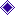Michael Coins LtdHomeEx-ShopAbout MichaelTermsGradingCode & RefGB Coin listExtras !British BanknotesOur Books, etcWorld CoinsWorld BanknotesAncientsOther ListsRecord & Video

COIN CLUBReading Coin Club

 Ref Type Series Date Denomination Prefix Grade Price L. K. O'BRIEN - 1st ISSUE - Britannia B273 L99J Start 1955 One Pound - L99J VF+/VF 9.50 B273 A~~K Mid 1955 One Pound 'First' - A47K EF+ 100.00 B273 A~~K Mid 1955 One Pound 'First' - A47K Run of 5 - Sold as pair or + (each) EF-EF+ 85.00 B273 A~~K Mid 1955 One Pound 'First' - A~~K EF-EF+ 18.50 B273 A~~K Mid 1955 One Pound 'First' - A~~K EF 14.00 B273 A~~K Mid 1955 One Pound 'First' - A~~K EF? 10.00 B273 A~~L Mid 1955 One Pound 'First' - A13L EF 14.00 B273 D01L Mid 1955 One Pound - D01L EF? 18.00 B273 l~~l Mid 1955 One Pound - S~~J Consecutive pair.   Sold as pair EF+ 38.00 B273 l~~l Mid 1955 One Pound - E~~K Consecutive pair.   Sold as pair EF+ 38.00 B273 l~~l Mid 1955 One Pound - R~~K Run of 4 - Sold as pair or + (each) EF+ 18.50 B273 l~~l Mid 1955 One Pound - S~~J; Y~~J - B~~K; D~~K - L~~K; X~~K - D~~L EF+ 17.50 B273 l~~l Mid 1955 One Pound - M~~J; O~~J - R~~J; S~~J - X~~J; Y~~J - D~~K; L~~K - M~~K; N~~K - J~~L EF-EF+ 12.50 B273 l~~l Mid 1955 One Pound EF 10.00 B273 l~~l Mid 1955 One Pound EF? 8.50 B273 l~~l Mid 1955 One Pound VF+ 7.50 B273 l~~l Mid 1955 One Pound VF+/VF 6.50 B273 l~~l Mid 1955 One Pound VF 5.50 B273 l~~l Mid 1955 One Pound VF/F 4.50 B273 Z~~J Mid 1955 One Pound 'Last' - Z~~J EF+ 27.50 B273 Z~~J Mid 1955 One Pound 'Last' - Z~~J EF 15.00 B273 Z~~J Mid 1955 One Pound 'Last' - Z~~J EF/EF? 10.00 B273 Z~~J Mid 1955 One Pound 'Last' - Z92J EF/VF+ 7.50 B273 Z~~K Mid 1955 One Pound 'Last' - Z~~K EF-EF+ 21.50 B273 Z~~K Mid 1955 One Pound 'Last' - Z~~K EF/EF? 10.00 1st ISSUE - REPLACEMENTS B274 S~~S Repl. 1955 One Pound - S96S EF+ 85.00 B274 S~~S Repl. 1955 One Pound - S81S F+ 12.50 B274 S~~T Repl. 1955 One Pound - S02T EF-EF+ 80.00 B274 S~~T Repl. 1955 One Pound - S14T EF 65.00 L. K. O'BRIEN - 2nd ISSUE - Portrait B281 A~~ Start 1960 One Pound - A77 Run of 4 - Sold as pair or + (each) EF+ 17.50 B281 A~~ Start 1960 One Pound - A~~ EF-EF+ 12.50 B281 A~~ Start 1960 One Pound - A6 EF 7.50 B281 A99 Start 1960 One Pound - A99 VF+/F+ 7.50 B281 l~~ Mid 1960 One Pound - B~~ Run of 13 - Sold as pair or + (each) EF+ 8.00 B281 l~~ Mid 1960 One Pound - H~~ Run of 9 - Sold as pair or + (each) EF+ 8.00 B281 l~~ Mid 1960 One Pound - L~~ Run of 28 - Sold as pair or + (each) EF+ 8.00 B281 l~~ Mid 1960 One Pound - J~~ EF+ 7.50 B281 l~~ Mid 1960 One Pound - B~~; J~~; K~~ - S~~; T~~ EF-EF+ 6.00 B281 l~~ Mid 1960 One Pound - B~~; D~~; J~~ EF 5.00 B281 l~~ Mid 1960 One Pound - H~~ EF? 4.50 B281 Z~~ End 1960 One Pound - Z~~ EF? 5.00 L. K. O'BRIEN - 2nd ISSUE - 2nd Type B282 ~~A Start 1961 One Pound - ~~A EF+ 16.50 B282 ~~A Start 1961 One Pound - ~~A EF-EF+ 11.50 B282 ~~l Mid 1961 One Pound - ~~L Run of 7 - Sold as pair or + (each) EF+ 8.50 B282 ~~l Mid 1961 One Pound - ~~N Consecutive pair.   Sold as pair EF+ 17.50 B282 ~~l Mid 1961 One Pound - ~~R Run of 4 - Sold as pair or + (each) EF+ 8.50 B282 ~~l Mid 1961 One Pound - ~~U Run of 7 - Sold as pair or + (each) EF+ 8.50 B282 ~~l Mid 1961 One Pound - ~~Y Run of 3 - Sold as pair or + (each) EF+ 8.50 B282 ~~l Mid 1961 One Pound - ~~K; ~~L EF+ 8.50 B282 ~~l Mid 1961 One Pound - ~~B; ~~C; ~~D - ~~J; ~~K; ~~L EF-EF+ 6.00 B282 ~~l Mid 1961 One Pound EF 5.00 B282 ~~l Mid 1961 One Pound EF? 4.50 B282 ~~Z End 1961 One Pound - 72Z Run of 3 - Sold as pair or + (each) EF+ 14.00 B282 ~~Z End 1961 One Pound - ~~Z EF+ 13.50 B282 ~~Z End 1961 One Pound - ~~Z EF-EF+ 10.00 B282 ~~Z End 1961 One Pound - ~~Z EF 7.50 L. K. O'BRIEN - 2nd ISSUE - 3rd Type B284 B01N Start 1962 One Pound -  'First' B01N VF+ 120.00 B284 B~~N Start 1962 One Pound - B~~N EF 18.00 2nd ISSUE - REPLACEMENTS B285 M15 Repl. 1960 One Pound - M15  (I Spy) Run of 8 - Sold as pair or + (each) EF+ 50.00 B285 M~~ Repl. 1960 One Pound - M~~ EF+ 38.50 B285 M~~ Repl. 1960 One Pound - M~~ Pin-head size rust mark - top left corner EF+ 30.00 B285 M~~ Repl. 1960 One Pound - M63 EF 22.50 B285 M~~ Repl. 1960 One Pound - M~~ 4 x 2mm size rust mark - top left corner EF+ 21.50 B285 M~~ Repl. 1960 One Pound - M~~ EF 20.00 B285 M~~ Repl. 1960 One Pound - M~~ EF? 15.00 B285 M~~ Repl. 1960 One Pound - M~~ VF+ 10.00 B285 M~~ Repl. 1960 One Pound - M~~ VF/F+ 6.50
 GB Banknote List O'Brien 10/- O'Brien £5